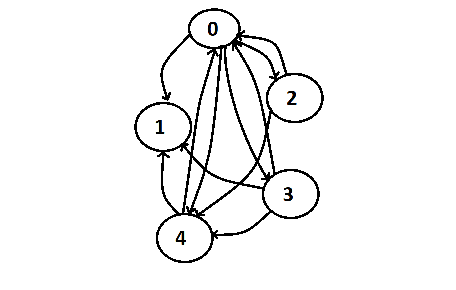### c++ boost::Graph::Graph_construction

Graphs are mathematical abstractions that are frequently used in computer science for solving many problems. Graph is one of the complicated data structure to implement. The Boost Graph Library provide us with already implemented graph functions in very generic form to be used without knowing its complicated implementation. The graph data structures are analogous to STL containers and Graph algorithms are analogous to STL algorithms. The graph abstraction consists of a set of vertices (or nodes), and a set of edges (or arcs) that connect the vertices.

The BGL(boost graph library) currently provides two graph classes, namely, adjacency_list and adjacency_matrix.

GRAPH CONSTRUCTION (using BGL)

In this section we will use adjacency_list class of BGL interface to construct a graph.

#include <utility>                   // for std::pair

using namespace boost;

typedef adjacency_list<vecS, vecS, bidirectionalS> Graph;   // typedef for the Graph type
typedef std::pair<int, int> Edge;

int main(int,char*[])
{

int ver = 5;        // number of vertices

Edge edge_array[] = { Edge(0,1), Edge(0,3), Edge(2,0), Edge(3,2), Edge(2,4), Edge(1,3), Edge(3,4) };

const int edges = sizeof(edge_array)/sizeof(edge_array);

Graph g(ver);      // declare a graph object

for (int i = 0; i < edges; ++i)

return 0;
}

*The adjacency_list is a template class with six
template parameters, though here we only fill in the first three parameters and
use the defaults for the remaining three.

*The first two template arguments (vecS,
vecS
) determine the data structure used to represent the out-edges for each
vertex in the graph. The third argument, bidirectionalS,
selects a directed graph that provides access to both out and in-edges. The
other options for the third argument are directedS which selects a
directed graph with only out-edges, and undirectedS which selects an
undirected graph.

* The above graph can be visualized as :